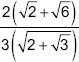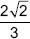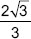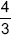# User Forum

Subject :IMO    Class : Class 10

The fractionis equal to _____.

ABCDMake the numerator in square root i.e. -/2 -/6 = -/ (8 4-/3) (( -/ is square root))Then numerator becomes the multiple of denominator of -/4 i.e. 2henve the answer becomes 4/3